# Class 9 Maths NCERT Solution for Heron Formula part 1

Notes Assignments Ncert Solutions Revision sheet

In this page we have NCERT book Solutions for Class 9th Maths:Heron Formula for EXERCISE 1 . Hope you like them and do not forget to like , social share and comment at the end of the page.
Question 1
A traffic signal board, indicating ‘SCHOOL AHEAD’, is an equilateral triangle with side ‘a’. Find the area of the signal board, using Heron’s formula. If its perimeter is 180 cm, what will be the area of the signal board?
Question 2:
The triangular side walls of a flyover have been used for advertisements. The sides of the walls are 122m, 22m, and 120m (see the given figure). The advertisements yield an earning of Rs 5000 per m2 per year. A company hired one of its walls for 3 months. How much rent did it pay?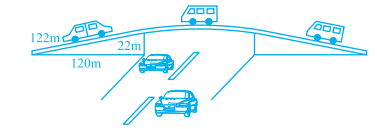Question 3
There is a slide in a park. One of its side walls has been painted in some color with a message “KEEP THE PARK GREEN AND CLEAN” . If the sides of the wall are 15 m, 11 m and 6 m, find the area painted in color.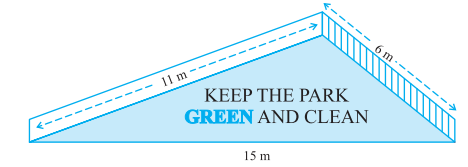Question 4:
Find the area of a triangle two sides of which are 18cm and 10cm and the perimeter is 42cm.
Question 5:
Sides of a triangle are in the ratio of 12 : 17 : 25 and its perimeter is 540cm. Find its area.
Question 6:
An isosceles triangle has perimeter 30 cm and each of the equal sides is 12 cm. Find the area of the triangle
Solution 1:
Semi-perimeter of triangle =s= (a+a+a)/2 =3a/2
Using Heron's formula,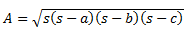Where  s is the semi-perimeter of triangle .
And, a, b and c are lengths of sides of triangle.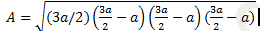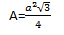If perimeter of equilateral triangle is equal to 180 cm then we can easily find length of each side.
Let length of each side =a cm.
According to the given condition, we have
a+a+a=180
3a=180
a=60 cm
Putting this value in Area formula we derived above, we get
Area of triangle =900√3 cm2

Solution 2:
Sides of triangle a, b, c are of 122 m, 22 m, and 120 m respectively
s = (122 + 22 + 120)/2 m
s = 132 m
By Heron's formulaA=1320 m2
Rent of 1 m2   area per year = Rs.5000
Rent of 1 m2 area per month = Rs 5000/12
Rent of 1320 m2 area for 3 months  = Rs.  (5000/12)X1320
= Rs.1650000
So, company had to pay Rs.1650000.
Solution 3:
It can be observed that the area to be painted in color is a triangle, having its sides as 11 m, 6 m, and 15 m.
s = (11 + 6 + 15)/2 m
s = 16 m
By Heron’s formula,A=20√2 m2
Therefore, the area painted in color is 20√2 m2
Solution 4:
a=18 cm
b=10 cm
Perimeter of triangle (a+b+c) =42 cm
c =42−18−10=14 cm
s = (18+10+14)/2=21cm
Using Heron's formula to find area of triangle, we getA=20√11 cm2
Solution 5:
Let the common ratio between the sides of given triangle be x.
So, side of triangle will be 12x, 17x, and 25x.
Perimeter of this triangle = 540 cm
12x + 17x + 25x = 540 cm
54x = 540 cm
x = 10 cm
Sides of triangle will be 120 cm, 170 cm, and 250 cm.
s=(a+b+c)/2=270 cm
By Heron's formulaA=9000 cm2
Solution 6:
Let the third side of this triangle be x.
Perimeter of triangle = 30 cm
12 cm + 12 cm + x = 30 cm
x = 6 cm
s=  30/2=15 cm
By Heron’s formula,Reference Books for class 9 Math

Given below are the links of some of the reference books for class 9 Math.

1. Mathematics for Class 9 by R D Sharma One of the best book for studying class 9 level mathematics. It has lot of problems to be solved.
2. Secondary School Mathematics for Class 9 by R S Aggarwal This is also as good as R.D. Sharma. Either this or the book by R.D. Sharma will do. I find book R.S. Aggarwal little bit more challenging than the one by R.D. Sharma.
3. Pearson IIT Foundation Series - Maths - Class 9 Buy this book if you want to challenge yourself further and want to prepare for JEE foundation.
4. Pearson IIT Foundation Physics, Chemistry & Maths combo for Class 9 Only buy if you are prepared to study extra topics and want to take your studies a step further. You might need help to understand topics in these books.

You can use above books for extra knowledge and practicing different questions.

Note to our visitors :-

Thanks for visiting our website.